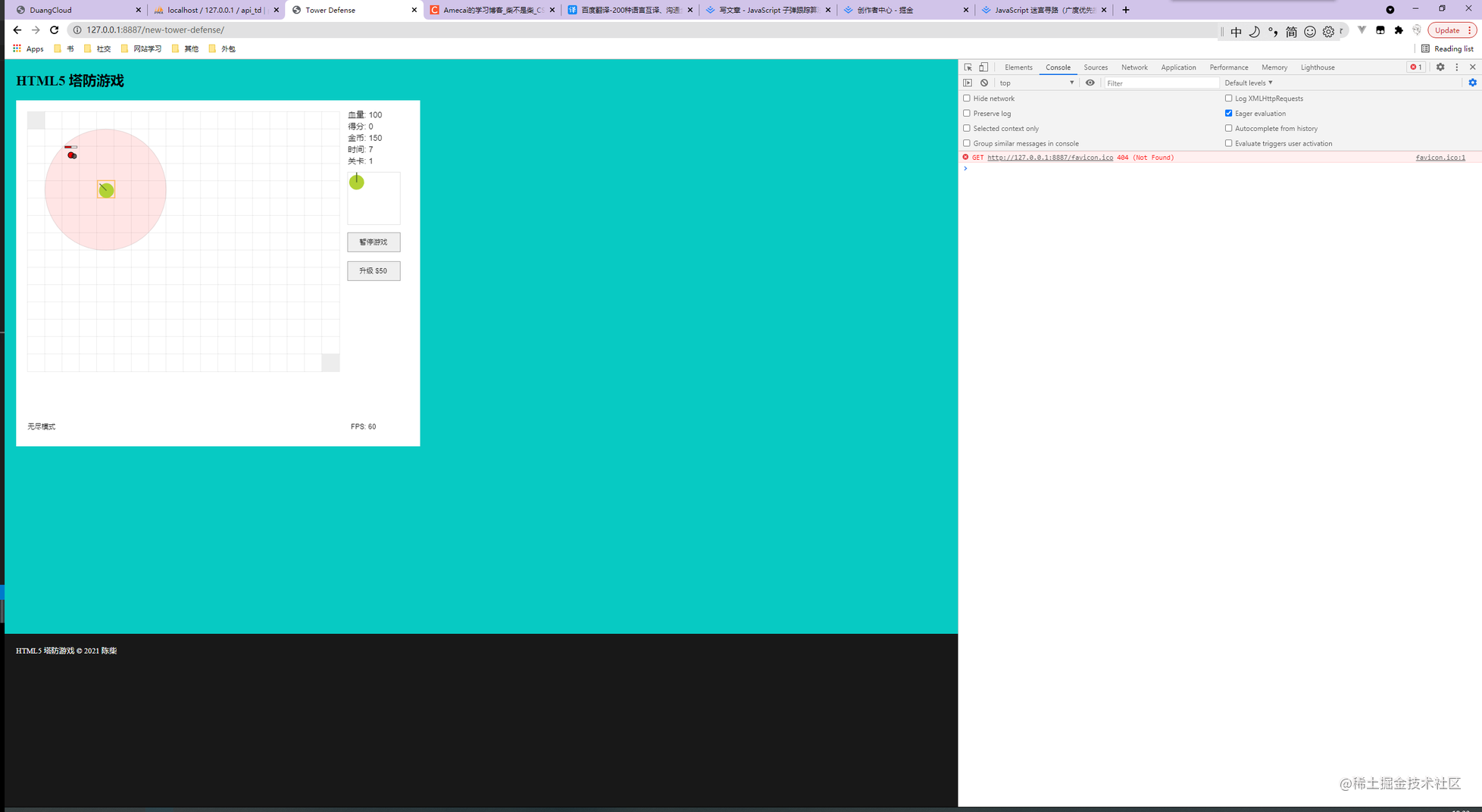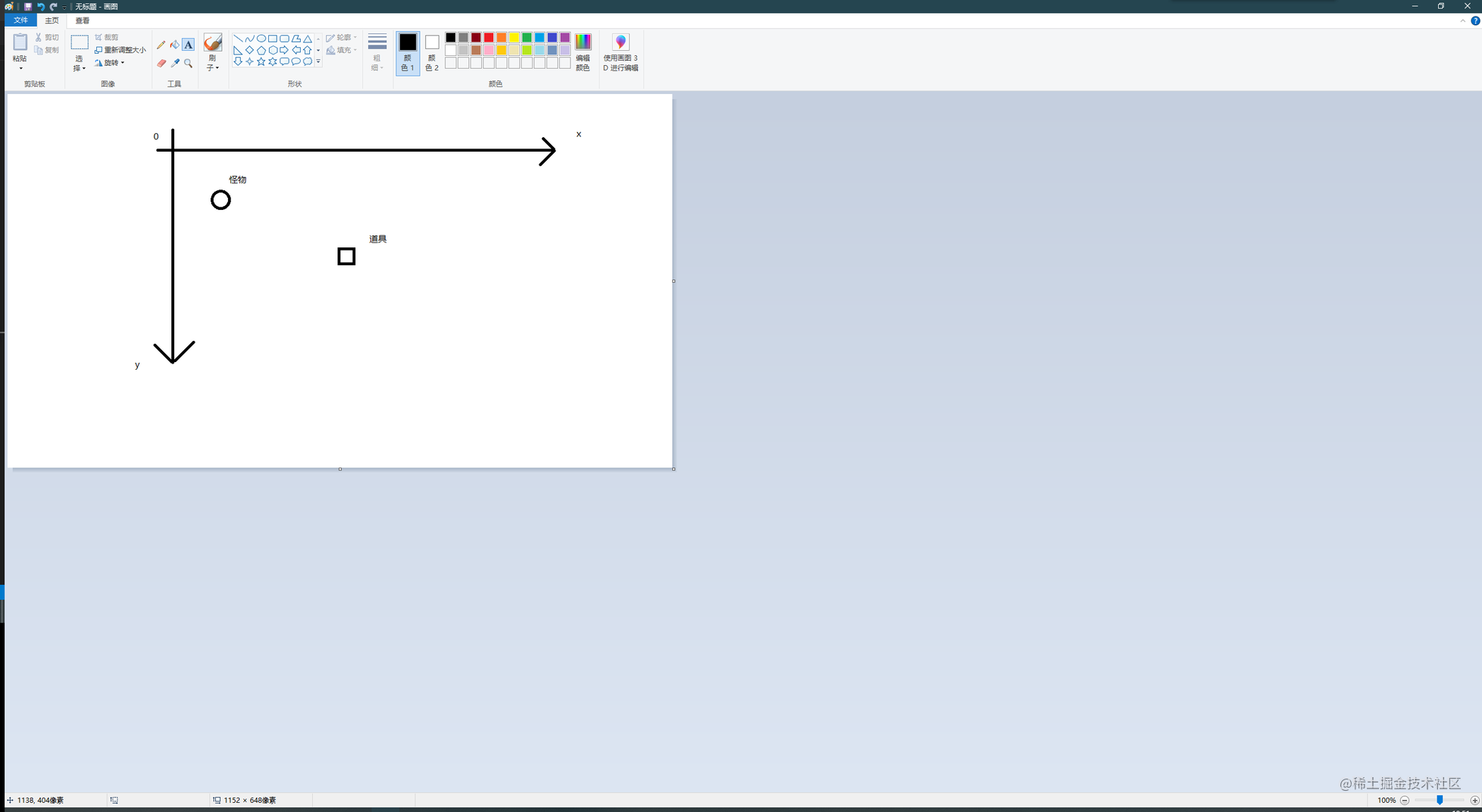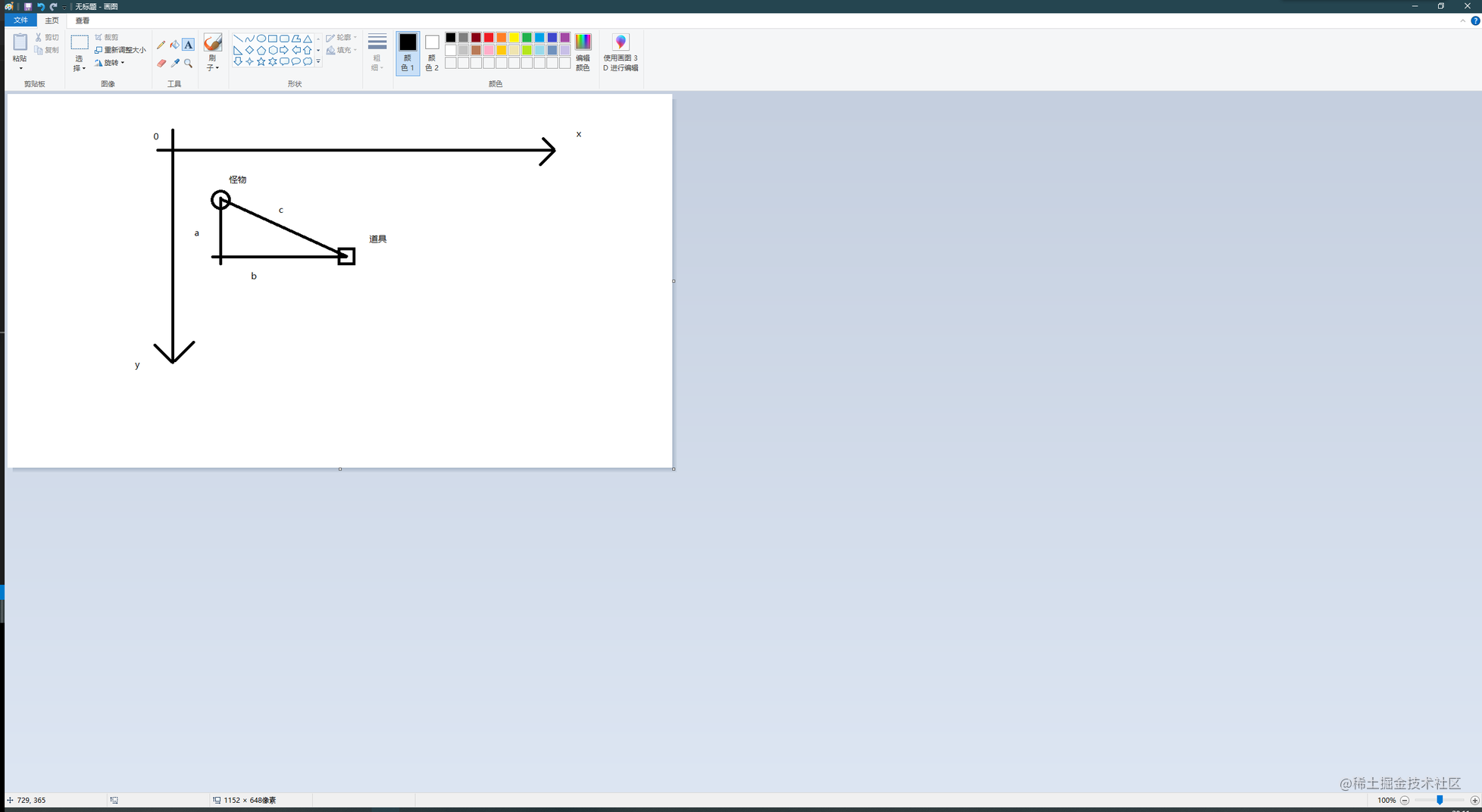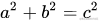# 一、实例截图# 二、功能分析

1. 子弹的速度是否会因怪物离道具的距离而发生变化？
2. 子弹需要如何移动？
3. 如何取得子弹的移动方向？x = 0, y = -1 则朝着正上方移动
x = 0, y = 1 则朝着正下方移动
x = -1, y = 0 则朝着正左方移动
x = 1, y = 0 则朝着正右方移动
x = 1, y = 1 则朝着右下方移动
x = 1, y = -1 则朝着右上方移动
x = -1, y = 1 则朝着左下方移动

# 三、勾股定理``````let x1 = 10,
x2 = 30,
y1 = 10,
y2 = 20,
c = Math.sqrt(Math.pow(Math.abs(x1 - x2), 2) + Math.pow(Math.abs(y1 - y2),2));// 22.360679774997898

``````const speed = 5;

``````let hy = c / speed;// 4.47213595499958

(不过在这里我们需要取反，不然的话子弹只会朝着相反的方向移动)

``````let x = -((x1 - x2) / hy),
y = -((y1 - y2) / hy);

``````const speed = 5;

function get_move_speed(m, t) {
let m_x = m.x + m.speed.x,
m_y = m.y + m.speed.y,
abs_x = Math.abs(t.x - m_x),
abs_y = Math.abs(t.y - m_y),
hy = Math.sqrt(Math.pow(abs_x, 2) + Math.pow(abs_y, 2)) / speed,
x = -((t.x - m_x) / hy),
y = -((t.y - m_y) / hy);

// 注意，这里 m 对象的坐标和 t对象的坐标 均是怪物与道具的中心点

return {x,y}
}

PS: 最后这里是做了点预判，因为怪物是会移动的，如果不做预判，很容易造成在子弹移动到怪物原来的位置后，怪物已经离开了。

（下一期或者下下期更新这个塔防游戏的如何实现~）。